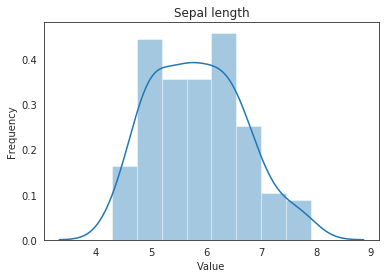# Summary of Seaborn Visualizations

Last updated: January 13th, 2020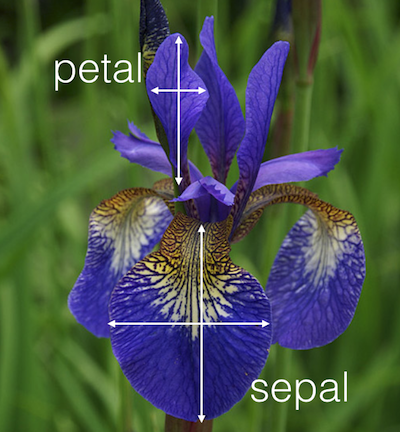# Summary of Seaborn visualizations¶

Far from being a visualization package created completely from scratch, the goal of seaborn has always been to offer simpler access to the creation of graphics based on matplotlib data. In fact, as indicated in its documentation, seaborn "should be seen as a complement to matplotlib and not a replacement of it."

seaborn depends on matplotlib for the own creation of graphics and only implements a layer of superior abstraction offers:

• Mapping of visual elements from full DataFrame used as input.
• Automation of certain statistical graphics.
• Topics and styles more visually attractive than matplotlib (at least in previous versions).
• Greater ease for the creation of "multigraphic" graphics.## Hands on!¶

In :
import numpy as np
import pandas as pd
import matplotlib.pyplot as plt
import seaborn as sns

%matplotlib inline

In :
iris = sns.load_dataset('iris')

In :
plt.scatter(iris['sepal_length'], iris['sepal_width'])

Out:
<matplotlib.collections.PathCollection at 0x7f2d1b023320>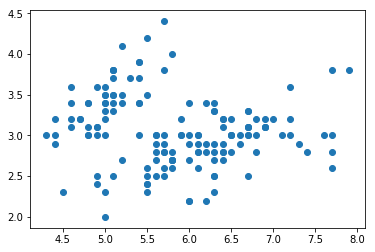### Some graphics with seaborn¶

Since seaborn is a complement to matplotlib, the types of graphics it offers are precisely those that matplotlib lacks (or complicates its use). However, since matplotlib continues to evolve, some of the graphics are already part of the library and, over time, it is expected that they will continue to be incorporated.

### One-variable distribution¶

As we have seen, one of the deficiencies that most impact when working with matplotlib is the possibility of showing the distribution of the variables (although we have histograms and, now, of the violin graphics).

Seaborn offers multiple functions that solve this lack for both univariate distributions:

In :
sns.distplot(iris['sepal_length'],
hist=True,
kde=True,
rug=True)

Out:
<matplotlib.axes._subplots.AxesSubplot at 0x7f2d1afd3f28>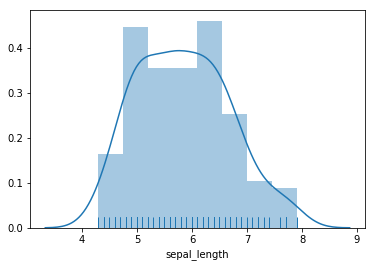In :
sns.kdeplot(iris['sepal_length'])

Out:
<matplotlib.axes._subplots.AxesSubplot at 0x7f2d1af1eb00>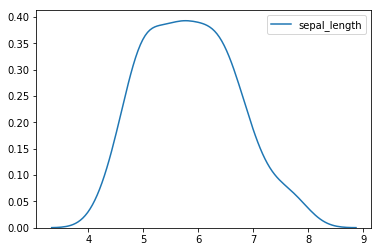In :
sns.rugplot(iris['sepal_length'])

Out:
<matplotlib.axes._subplots.AxesSubplot at 0x7f2d1af80e10>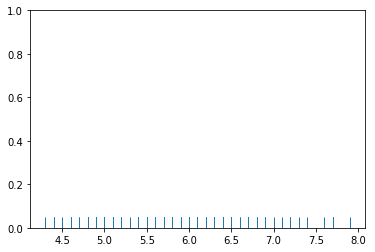### Two-variable distribution¶

Also for bivariate distributions:

In :
sns.jointplot(x='sepal_length', y='sepal_width', data=iris,
kind='scatter')

Out:
<seaborn.axisgrid.JointGrid at 0x7f2d1ae0ce80>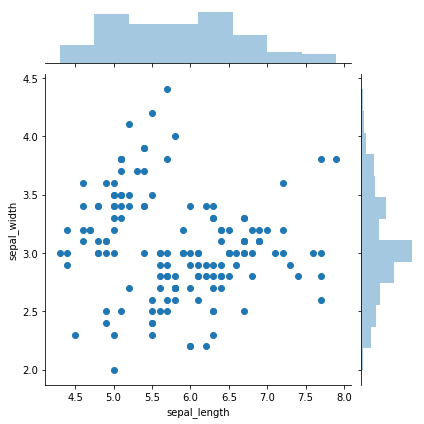In :
sns.jointplot(x='sepal_length', y='sepal_width', data=iris,
kind='hex')

Out:
<seaborn.axisgrid.JointGrid at 0x7f2d1ad56630>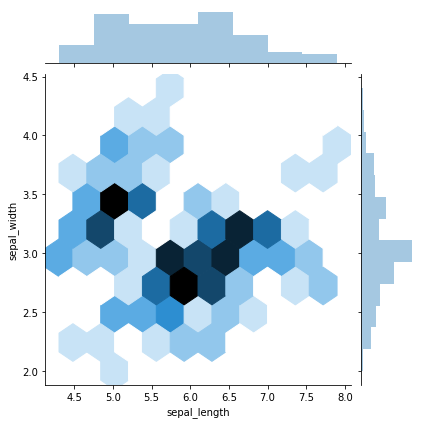In :
sns.jointplot(x='sepal_length', y='sepal_width', data=iris,
kind='kde')

Out:
<seaborn.axisgrid.JointGrid at 0x7f2d1ac10c50>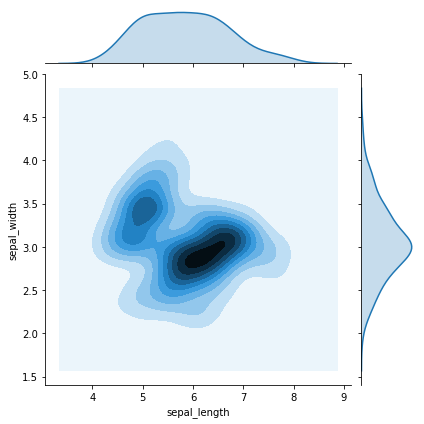### Pairplots, relating two or more variables¶

These plots are used to study the relationship between all the variables with a single visualization.

In :
sns.pairplot(iris)

Out:
<seaborn.axisgrid.PairGrid at 0x7f2d1aacdbe0>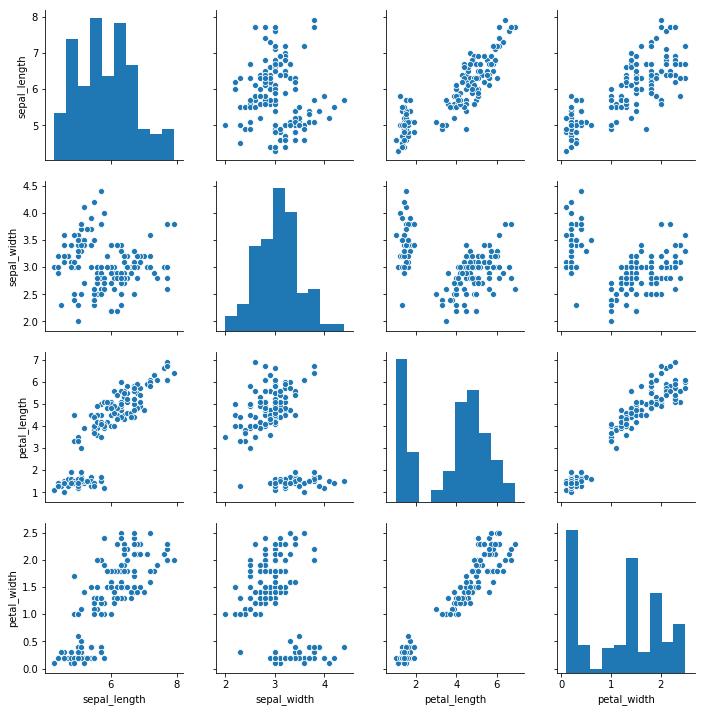In :
sns.pairplot(iris, vars=['sepal_width', 'petal_length'])

Out:
<seaborn.axisgrid.PairGrid at 0x7f2d1a51cf28>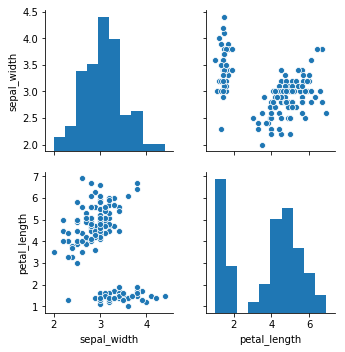In :
sns.pairplot(iris, diag_kind='kde')

Out:
<seaborn.axisgrid.PairGrid at 0x7f2d1a362c18>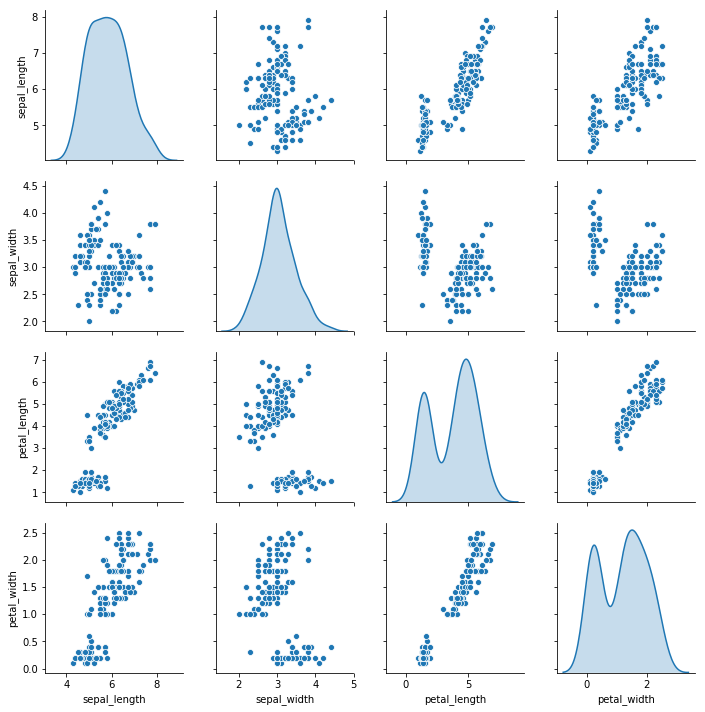### Regression Plots¶

Another of the weak points of matplotlib is the inclusion of "statistics" that help to understand the content of a visualization and its underlying dataset directly (since they can always be calculated and included as an additional layer to any graphic).

Seaborn tries to include this functionality to "approximate" that stats.

In :
sns.regplot(x='sepal_length', y='sepal_width', data=iris)

Out:
<matplotlib.axes._subplots.AxesSubplot at 0x7f2d13f3af28>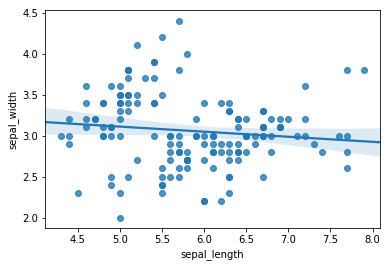That doesn't look too good. Regressions are used to analyze the correlation between different variables. If there's a strong correlation, the points will be aligned in a diagonal.

Let's create a new column which makes this relationship a little bit more clear:

In :
iris['Sepal Area'] = iris['sepal_length'] * iris['sepal_width']

In :
sns.regplot('sepal_length', 'Sepal Area', data=iris)

Out:
<matplotlib.axes._subplots.AxesSubplot at 0x7f2d13c98390>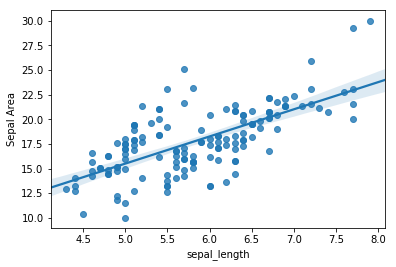There's also a lmplot, which lets you specify "polynomial regressions" (this will make more sense when we start talking about Machine Learning in the future):

In :
sns.lmplot(data=iris, x='sepal_length', y='Sepal Area',
order=3)

Out:
<seaborn.axisgrid.FacetGrid at 0x7f2d13cdabe0>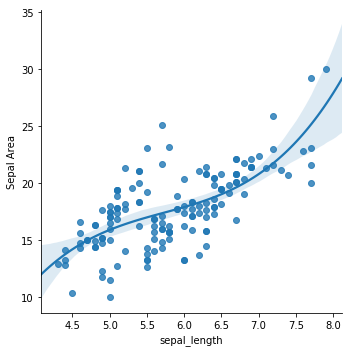In :
sns.lmplot(data=iris, x='sepal_length', y='Sepal Area',
hue='species',
markers=["+", "o", "^"])

Out:
<seaborn.axisgrid.FacetGrid at 0x7f2d13befbe0>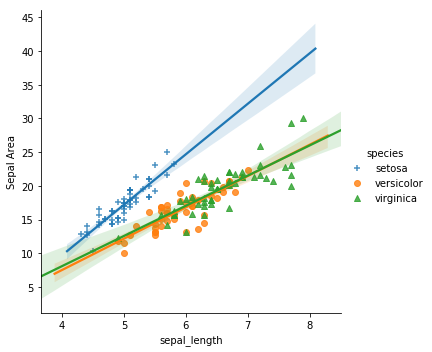In :
del iris['Sepal Area']### Charts based on categorical variable¶

We have seen that matplotlib treats ALL the variables included in the charts as numerical sets. If you want to make visualizations based on categorical variables, you need to work with them as numeric and "trick" the content of the axes to give the "feeling" of working with numerical variables.

Seaborn allows the direct use of categorical variables and their correct treatments.

In :
sns.stripplot(data=iris, x='species', y='sepal_length')

Out:
<matplotlib.axes._subplots.AxesSubplot at 0x7f2d13c7a240>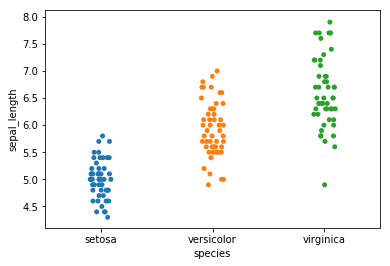In :
sns.stripplot(data=iris, x='sepal_length', y='species',
jitter=False)

Out:
<matplotlib.axes._subplots.AxesSubplot at 0x7f2d13b23da0>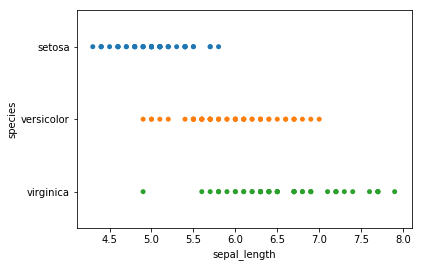In :
sns.stripplot(data=iris, x='sepal_length', y='sepal_width',
hue='species')

Out:
<matplotlib.axes._subplots.AxesSubplot at 0x7f2d13af4198>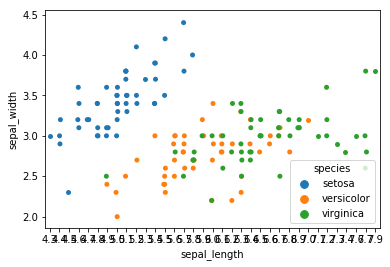In :
sns.swarmplot(data=iris, x='species', y='sepal_length')

Out:
<matplotlib.axes._subplots.AxesSubplot at 0x7f2d13a10ac8>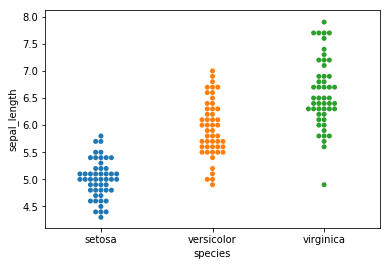In :
sns.boxplot(data=iris, x='species', y='sepal_length')

Out:
<matplotlib.axes._subplots.AxesSubplot at 0x7f2d13957dd8>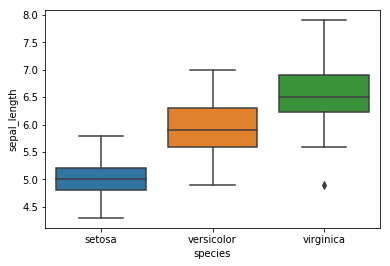In :
sns.violinplot(data=iris, x='species', y='sepal_length')

Out:
<matplotlib.axes._subplots.AxesSubplot at 0x7f2d138dbac8>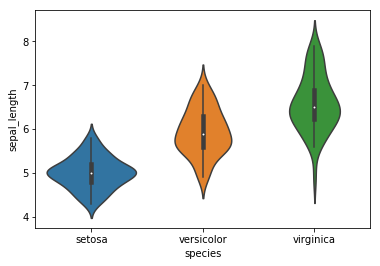### Multigraphic with seaborn¶

Although we have seen that matplotlib allows the creation of multigraphic graphics, we have also talked about the limitations of its generation since they are based solely on positioning and it is the responsibility of the user to adjust scales, the drawing of each graphic in its corresponding position, etc.

In :
grid = sns.FacetGrid(iris, col='species', hue='species')

grid.map(plt.scatter,'sepal_length', 'sepal_width')

Out:
<seaborn.axisgrid.FacetGrid at 0x7f2d138cc8d0>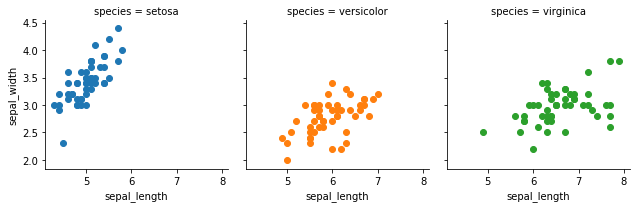Also, a legend can be added to the grid plots:

In :
grid = sns.FacetGrid(iris, col='species', hue='species')

grid.map(plt.scatter,'sepal_length', 'sepal_width')


Out:
<seaborn.axisgrid.FacetGrid at 0x7f2d137d2160>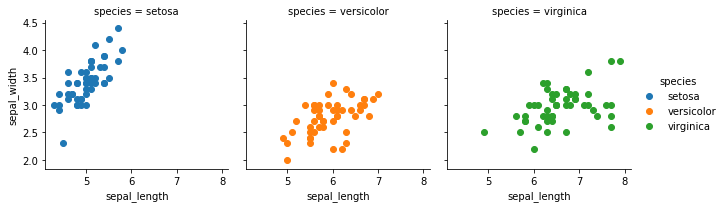In :
diamonds = pd.read_table('data/diamonds.txt', sep=',')

grid = sns.FacetGrid(diamonds, col='cut', row='color')

grid.map(plt.hist, 'price')

Out:
<seaborn.axisgrid.FacetGrid at 0x7f2d136c55c0>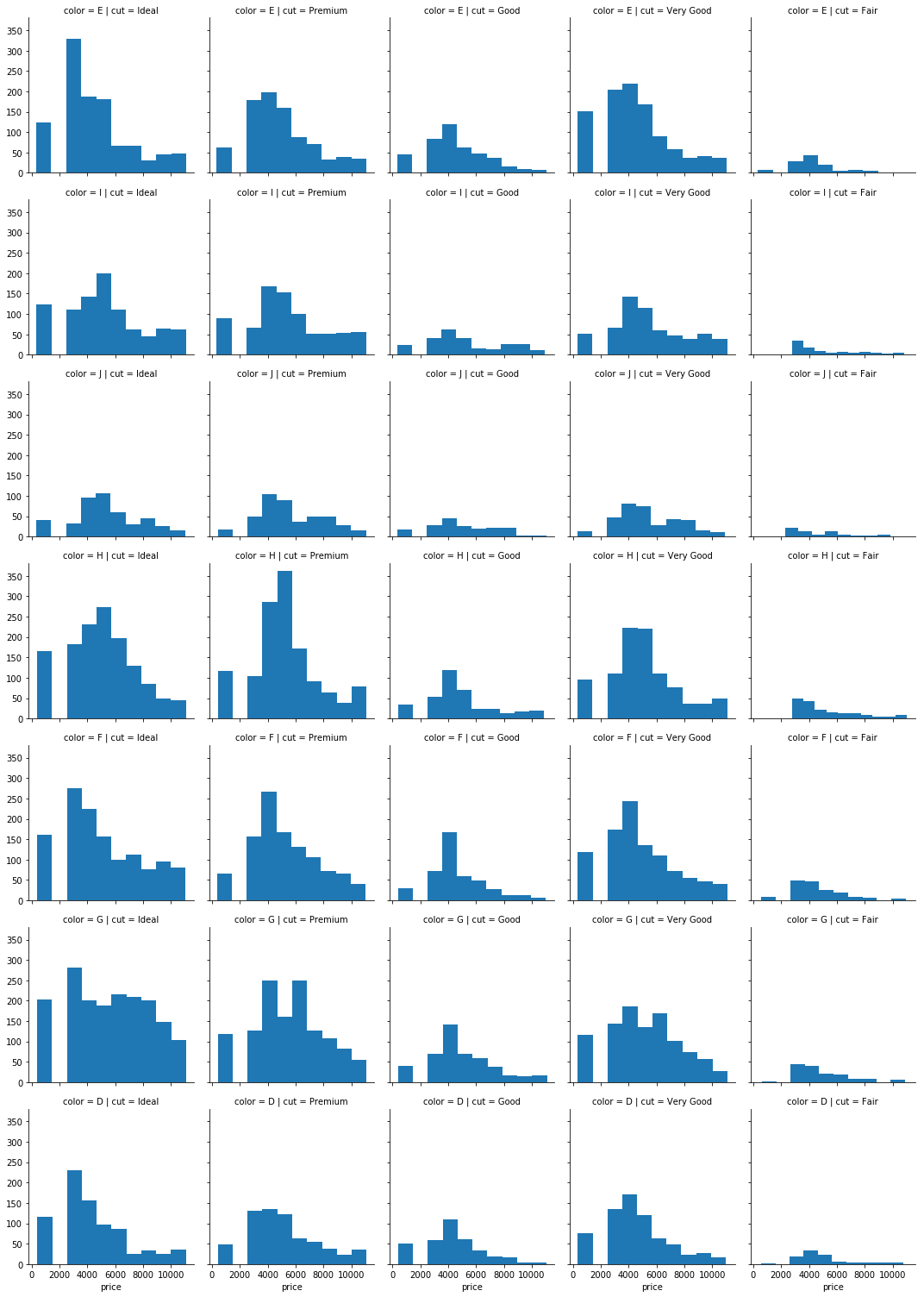### Styles in seaborn¶

At the time of the creation of seaborn, the style management of matplotlib was very limited and "forced" its definition based on matplotlibrc files (costly, uncomfortable, inflexible and a priori).

Currently matplotlib has improved a lot in this aspect and the "advantages" offered by seaborn are not so clear.

In :
sns.axes_style()

Out:
{'axes.facecolor': 'w',
'axes.edgecolor': 'k',
'axes.grid': False,
'axes.axisbelow': 'line',
'axes.labelcolor': 'k',
'figure.facecolor': (1, 1, 1, 0),
'grid.color': '#b0b0b0',
'grid.linestyle': '-',
'text.color': 'k',
'xtick.color': 'k',
'ytick.color': 'k',
'xtick.direction': 'out',
'ytick.direction': 'out',
'lines.solid_capstyle': 'projecting',
'patch.edgecolor': 'k',
'image.cmap': 'viridis',
'font.family': ['sans-serif'],
'font.sans-serif': ['DejaVu Sans',
'Bitstream Vera Sans',
'Computer Modern Sans Serif',
'Lucida Grande',
'Verdana',
'Geneva',
'Lucid',
'Arial',
'Helvetica',
'Avant Garde',
'sans-serif'],
'patch.force_edgecolor': False,
'xtick.bottom': True,
'xtick.top': False,
'ytick.left': True,
'ytick.right': False,
'axes.spines.left': True,
'axes.spines.bottom': True,
'axes.spines.right': True,
'axes.spines.top': True}
In :
sns.regplot(data=iris, x='sepal_length', y='sepal_width')

Out:
<matplotlib.axes._subplots.AxesSubplot at 0x7f2d127a2358>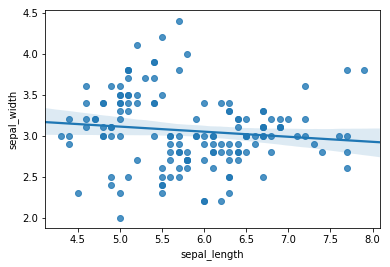In :
sns.set_style('white')

sns.regplot(data=iris, x='sepal_length', y='sepal_width')

Out:
<matplotlib.axes._subplots.AxesSubplot at 0x7f2d11acd780>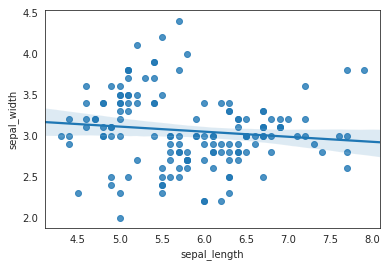In :
sns.set_style('darkgrid', {
"grid.color": "#cccccc",
"grid.linestyle": "--",
"figure.facecolor": "#eeeeee"})

sns.regplot(data=iris, x='sepal_length', y='sepal_width')

Out:
<matplotlib.axes._subplots.AxesSubplot at 0x7f2d11a8d6a0>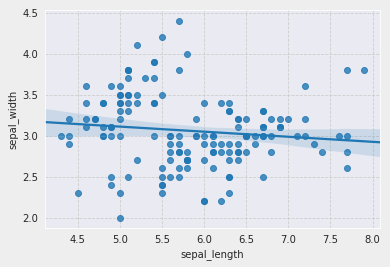Top and right borders can be removed using the dispine() function.

In :
sns.set_style("white")

sns.distplot(iris['sepal_length'])

sns.despine()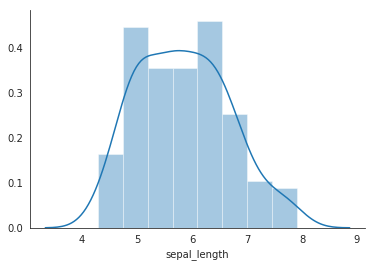You can also modify title and axis labels in Seaborn.

In :
sns.distplot(iris['sepal_length'])

Out:
<matplotlib.axes._subplots.AxesSubplot at 0x7f2d119c4ba8>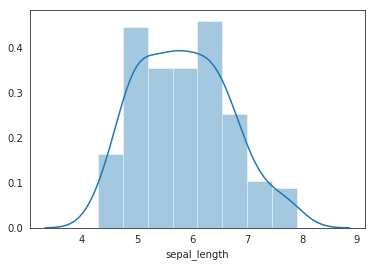In :
plot = sns.distplot(iris['sepal_length'])

plot.set(title='Sepal length',
xlabel='Value',
ylabel='Frequency')

Out:
[Text(0,0.5,'Frequency'), Text(0.5,0,'Value'), Text(0.5,1,'Sepal length')]